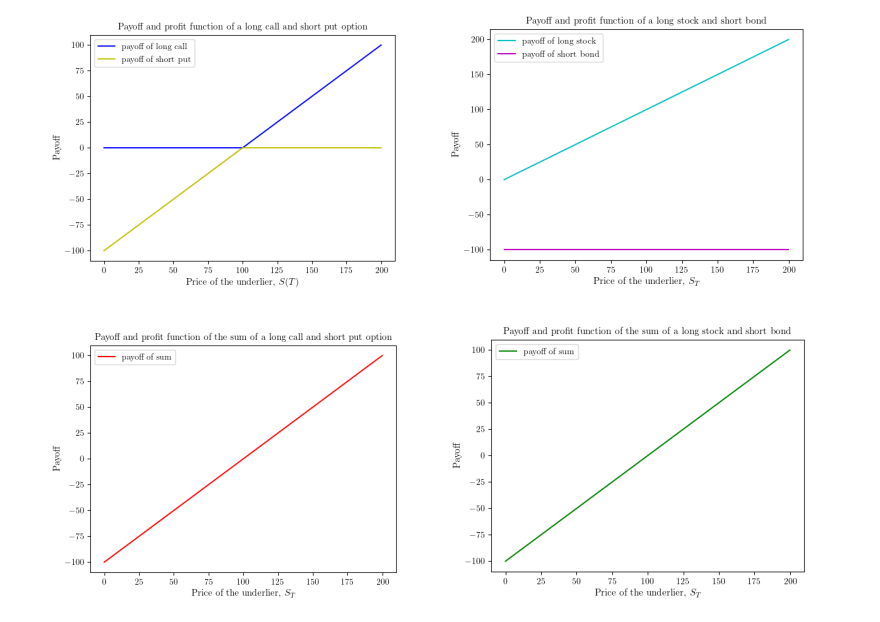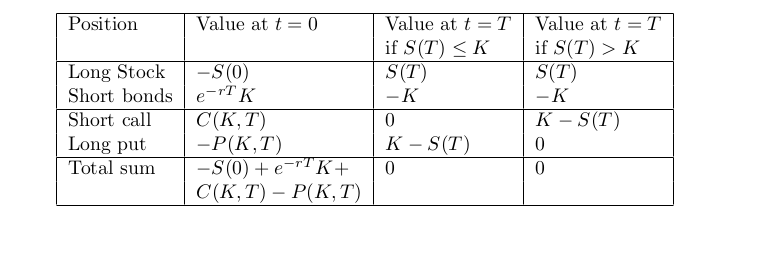📓

# Put-call parity

In this article, we explore a relationship between the price of a European call option and a European put option that both have the same underlier, expiry, and strike price. This knowledge can help you identify arbitrage opportunities in an options market if the put-call parity isn’t maintained, as well as pin down prices for a put given a call price or vice-versa.

We’ll first discuss some aspects of interest rates that we will need in this and future articles.

# Compounding Interest Rates

Simple interest rates do not involve the compounding of money. For example, if \$100 is invested at an annual interest rate of 6 percent, it grows to \$106 a year from now. Compound interest is when interest is earned on the principal plus any accrued interest. For example, if the compounding interval is 1 month, with an annual rate of 6 percent, \$100 grows to 100 + 6/12 = \$100.5 in a month. In a year, there are 12 such compounding periods, so it grows to

\$100*(1+6/12)¹² = \$106.16.

In general, if the interest rate is r (in decimals, so 6 percent means r = 0.06), and there are m compounding periods in a year, the principal grows to (1+r/m)ᵐ times the principal in a year. If the interest is continuously compounded, that is, we let m become very large, the expression (1+r/m)ᵐ approaches the exponential , where e is a special mathematical constant that is roughly 2.71828. In our previous example, exp(0.06)= 1.0618. In general, if continuous compounding at the constant rate of r occurs until time t, we have exp(rt).

# Zero Coupon Bonds

A zero coupon bond(ZCB) with face value (par value) K and maturity T is an interest bearing instrument in which an investor can pay a discounted price B₀ relative to par value today to purchase the right to earn at time T. This is also sometimes called a money market account. Assuming a constant continuously compounded risk-free rate r, the price of a ZCB today is B₀ = K*exp(-rT).

# Put-Call Parity

Now to return to the previously scheduled option strategy content. Something magical happens when we buy (long) a call option, and write (short) a put option, with the same strike price and expiration:In this case, we have a portfolio consisting of a long call option with a strike price of 100 and a short put option with a strike price of 100 (shown in the left column), contrasted with a portfolio consisting of that stock with a short bond with a future liability of 100 (shown on the right column). Notice that when added, both portfolios give the same payoff. We can write this as:

Long call + short put = long stock + short bond

Rearranging the terms in this equation also yields another interesting relationship:

Long stock + short call = short put + long bond

In words, a covered call = cash secured put, where the put and call have the same strike and expiration.

We can also represent this using a table:In the above portfolio, we’ve moved the long call and short put on the left hand side of the equation above to the right hand side. Notice that at time t = T, the portfolio is always worth S(T)-K + K -S(T) = 0, regardless of whether S(T) is greater than or less than K. So, the portfolio must be worth 0 initially (at time t=0), otherwise we have an arbitrage opportunity. This allows us to write put-call parity in the following form, which is the same as the relationship obtained from the payoff diagram above (note that a long call has the negative payoff of a short call):

Let’s consider an example to see how this relationship can be used:

• A stock costs S(0) at t = 0, for example, let this be \$112.5. We represent its future price at time t= T as S(T)
• Let the current compounded interest rate to be 5 percent a year, and let T be one year, so that exp(-0.05*1) = 0.95. Note that the shorted bond needs to be repaid with amount K = 100 after one year. Short selling the bond today (i.e. borrowing it to sell), gives us 100*exp(-0.05*1).
• The put costs p today. (If S(T) < K at expiration, then the put pays K-S(T)).
• The call costs c today. (If S(T) > K at expiration, the short call is worth K-S(T))
• Suppose the price of a put today is \$2.5, now we can determine the arbitrage-free price of the call.

Set c = S(0) - K*exp(-rT) + p = 112.5-0.95*100 + 2.5 = 20, which implies the arbitrage free price for the call option is \$20.

# Next Time

In our next article, we will look into Greeks, which capture rates of change for options prices with respect to various factors , and which will be necessary for us to explain the Arrow protocol in more detail.